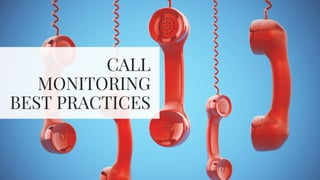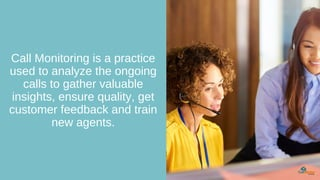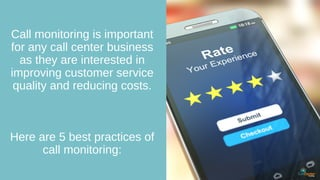Successfully reported this slideshow.

# Call Monitoring - 5 Best Practices×

# Call Monitoring - 5 Best Practices

Call monitoring is an easy and simple process that will generate good results for a business.
Customer experience is a prime factor for any business.
Cloud-based call center with the call monitoring feature helps in analyzing all the calls to improve customer service and further lead to better customer experience.
Here are 5 best practices of call monitoring.

Call monitoring is an easy and simple process that will generate good results for a business.
Customer experience is a prime factor for any business.
Cloud-based call center with the call monitoring feature helps in analyzing all the calls to improve customer service and further lead to better customer experience.
Here are 5 best practices of call monitoring.

### Call Monitoring - 5 Best Practices

1. 1. CALL MONITORING BEST PRACTICES
2. 2. Call Monitoring is a practice used to analyze the ongoing calls to gather valuable insights, ensure quality, get customer feedback and train new agents.
3. 3. Call monitoring is important for any call center business as they are interested in improving customer service quality and reducing costs. Here are 5 best practices of call monitoring:
4. 4. C a l l c e n t e r m a n a g e r s m u s t h e a r t h e e n t i r e c o n v e r s a t i o n b e t w e e n t h e c u s t o m e r a n d t h e a g e n t t o a n a l y z e t h e c a l l q u a l i t y a n d p e r f o r m a n c e o f a n a g e n t . 1 . O B S E R V E E N T I R E C A L L E R E X P E R I E N C E
5. 5. A g e n t s m u s t b e p r o v i d e d w i t h s o m e t i m e t o a n a l y z e t h e i r p e r f o r m a n c e a n d f u r t h e r t a k e s t e p s t o i m p r o v e t h e q u a l i t y o f a c a l l . 2 . A L L O W A G E N T S E L F - A S S E S S M E N T
6. 6. C a l l c e n t e r m a n a g e r s s h o u l d l i s t e n t o m o s t o f t h e c a l l s . T h e y m u s t p r i o r i t i z e t h e c a l l s h a n d l e d b y t h e a g e n t s s u c h a s p o t e n t i a l c u s t o m e r s , r e c o r d i n g s w i t h m i n i m a l i n t e r a c t i o n , c u s t o m e r s w i t h a b a d e x p e r i e n c e , e t c . 3 . O P T I M I Z E C A L L S E L E C T I O N
7. 7. A g e n t s m u s t b e t r a i n e d w e l l t o r e s o l v e c u s t o m e r q u e r i e s i n l e s s t i m e f o r i m p r o v i n g c u s t o m e r s a t i s f a c t i o n . 4 . F O C U S O N A G E N T C O A C H I N G
8. 8. M a n a g e r s s h o u l d a s s i g n d i f f e r e n t a g e n t s t o b e a p a r t o f a s p e c i f i c c a l l m o n i t o r i n g t e a m t o r u n t h e p r o c e s s s m o o t h l y . 5 . D E S I G N A C A L L Q U A L I T Y M O N I T O R I N G T E A M
9. 9. About Us CallCenterHosting (CCH) is a leading and globally recognized provider of call center solutions. We offer a complete software-based call center set up for start-ups, SMBs, and enterprise, which includes call center dialers such as- predictive dialer, robo dialer, power dialer, and progressive dialer, IVR services, outbound & inbound call center services and For more information, call us at +1-800-346-4974 auto dialer.
10. 10. Liked Us? Find Similar Posts Here... Follow Us For More Content
11. 11. References The 5 Steps to Improve Your Call Quality Monitoring